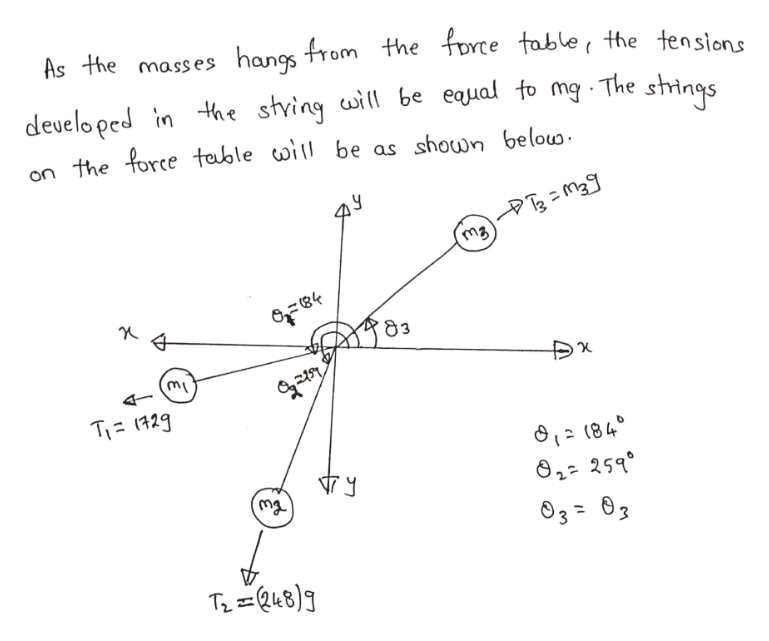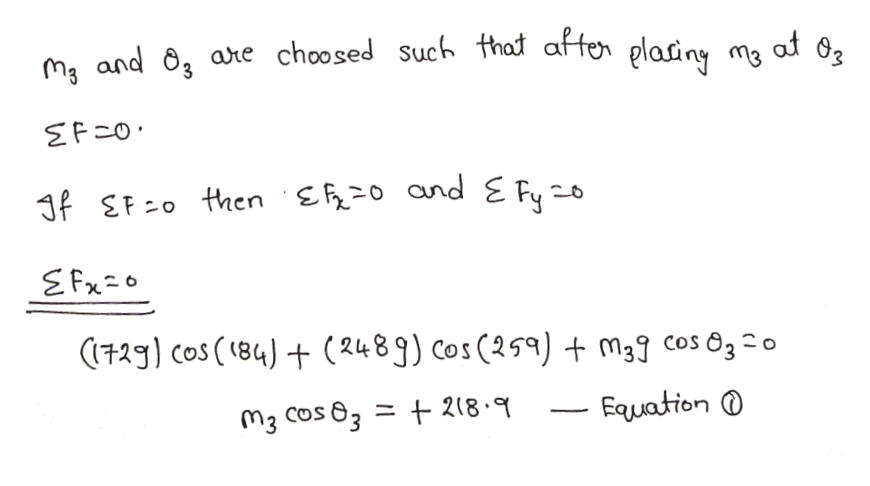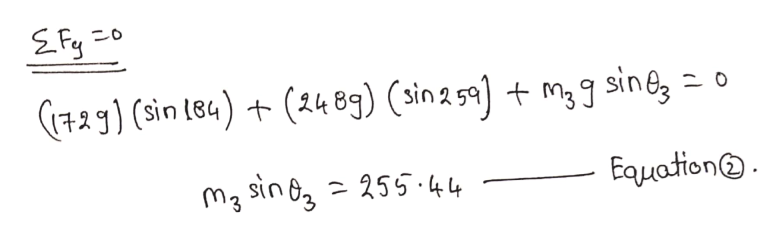A student hangs three masses from a force table. If the first mass of 172g is at an angle of 184 degrees, and she then hangs a mass of 248g at 259 degrees, calculate the mass and angle of the third mass so that ring on the force table is in equilibrium. Assume the given masses include the mass of the hangers.

Question

A student hangs three masses from a force table. If the first mass of 172g is at an angle of 184 degrees, and she then hangs a mass of 248g at 259 degrees, calculate the mass and angle of the third mass so that ring on the force table is in equilibrium. Assume the given masses include the mass of the hangers.

Step 1help_outlineImage TranscriptioncloseAs the mass es hangs from the force table the tensions the string wil be eaual to mg The strings develo ped in on the force table wi be as shown belouo m3 4- TI 129 (84 02 259 03=03 T48) fullscreen
Step 2help_outlineImage Transcriptioncloseare choosed Such that affen plasiny ma maand at 3f EF o then ER2o and E Fyo EFxo 729 cos (184t (2489) Cos(259) + m3? cos 632a 218- Fauation M3 Cos 63 fullscreen
Step 3help_outlineImage TranscriptioncloseC()Sin tea)(4 8g) (sin2 52) + m3g sine o Σ0 Eομαίοη@ . m3 sin 255.44 fullscreen

Want to see the full answer?

See Solution

Want to see this answer and more?

Our solutions are written by experts, many with advanced degrees, and available 24/7

See Solution
Tagged in

Physics